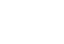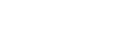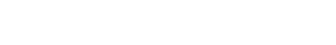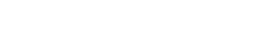AP Calculus BC Review: Parametric Functions

Parametric functions only show up on the AP Calculus BC exam. In this article we’ll take a close look at these kinds of functions which turn out to be extremely useful in the sciences.

In fact, this is one case in which the phrase “It’s not rocket science!” isn’t really appropriate. Parametric equations play a huge role in rocket guidance systems!The path of a rocket can be modeled using parametric functions.

What are Parametric Functions?

While they may at first seem foreign and confusing, parametric functions are just a more flexible way to track motion in the plane.

Usually you might think of a function as y = f(x). The graph of a function must pass the Vertical Line Test (VLT), and as a result, your options are limited.

Sure you can use typical functions to define parabolas, catenaries and even sinusoidal waves, but what about a the orbit of a planet?

Certainly any circular or elliptical graph fails the VLT. So you can’t write a function of the form y = f(x) for this situation.The graph of an ellipse is not a function of the form y = f(x). Instead, you could use the parametric function defined by x = 3 cos(t) and y = 5 sin(t).

Instead, you need to be able to specify both x and y in terms of some independent parameter, t. That’s where the term parametric comes from.

Definition

A parametric function (or a set of parametric equations) is a pair of two functions specifying the x– and y-coordinates of a point moving through the plane.Think of each function as a separate control, one for x and one for y. Perhaps the best physical example of parametric equations is the Etch-A-Sketch.

The Etch-A-Sketch has two knobs, one controlling the vertical, and one controlling the horizontal position of a stylus. When the two knobs work together, almost anything can be drawn!This is about the best I could ever manage on an Etch-A-Sketch.

Graphing

There is a straightforward way to graph any parametric function.

1. Choose a number of sample t-values. If there is a given range of values, atb, then you must stick to values within that interval. Otherwise, just pick a wide range of values, both positive and negative.
2. For each sample t, plug it into x = f(t) and into y = g(t) to find out the corresponding x– and y-coordinates.
3. Plot each (x, y) pair on the plane. Then connect the dots in the order of increasing t.

Example Graph

Graph the parametric function defined by x = t2 – 2t + 1 and y = –t2 + 2.

Because there was no range specified for t, let’s just pick a few easy numbers to work with. Remember, use both positive and negative values to get a good sense for how the function behaves.

I like to organize my work in a table.

tx = t2 - 2t + 1y = -t2 + 2(x, y)
-316-7(16, -7)
-29-2(9, -2)
-141(4, 1)
012(1, 2)
101(0, 1)
21-2(1, -2)
34-7(4, -7)

Next, plot these points on a coordinate plane.Finally, connect the dots in order of increasing t.Derivatives of Parametric Functions

Now that you have seen some graphing, let’s talk about slope. As you know, the derivative measures slope. But how do you find the derivative of a set parametric equations?

We must be careful, because there are two equations to deal with. Should you take the derivative of f(t) or g(t)?

In fact, you’ll have to take the derivative of both. Here is the formula for dy/dx.Example — Tangent Line

What is the equation of the tangent line at t = π/6 for the parametric function x = 3 cos t, y = 3 sin t?

Using the derivative formula, we get:

dy/dx = (3 cos t)/(-3 sin t) = -cos t / sin t.

Plugging in t = π/6, the slope is -cos(π/6)/sin(π/6) = -1.732.

Now we also need to know what the x– and y-coordinates are for the point in question.

x = 3 cos(π/6) = 2.598, and y = 3 sin(π/6) = 1.5.

Therefore, using the point-slope form, the equation of the tangent line is:

y = -1.732(x – 2.598) + 1.5

Derivatives and Velocity

There is another interpretation of the derivative that allows you to compute the velocity of an object traveling along a parametric curve.

The idea is to think of a parametric function as a vector.

Thinking of t as time, then both the velocity vector and accelerations vectors are simply derivatives in each component.Example — Velocity

Find the velocity vector at t = 1 for an object traveling according to the parametric function x = t2 – 2t + 1, y = –t2 + 2.

First find the derivative of each component function.

v(t) = (2t – 2, -2t).

Therefore at time t = 1, the velocity vector is:

v(1) = (0, -2).

Length Integrals

Another important formula to memorize is the arc-length integral. If you want to know the length of the curve traced out by the parametric function x = f(t), y = g(t), for atb, then just set up and compute the following integral.Example — Length of a Parametric Curve

Find the length of the curve defined by x = 3 cos t, y = 3 sin t on the interval [0, π/2].Summary

Parametric functions show up on the AP Calculus BC exam. You should know the following.

• How to graph or interpret the graph of a parametric function
• Finding the slope at any given point on a parametric curve
• Computing the velocity vector
• Finding the length of the curve

Improve your SAT or ACT score, guaranteed. Start your 1 Week Free Trial of Magoosh SAT Prep or your 1 Week Free Trial of Magoosh ACT Prep today!Author

•Shaun earned his Ph. D. in mathematics from The Ohio State University in 2008 (Go Bucks!!). He received his BA in Mathematics with a minor in computer science from Oberlin College in 2002. In addition, Shaun earned a B. Mus. from the Oberlin Conservatory in the same year, with a major in music composition. Shaun still loves music -- almost as much as math! -- and he (thinks he) can play piano, guitar, and bass. Shaun has taught and tutored students in mathematics for about a decade, and hopes his experience can help you to succeed!